Self Inductance

Whenever you change the current in a coil of wire, the magnetic field it produces will change. That will change the magnetic flux through the coil and hence produce a voltage across the coil. This phenomenon is called self-inductance, and the coil is referred to as an inductor.

The quantitative measure of this effect is the inductance L defined by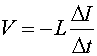An inductor can store energy. The power (= energy / time) being stored in an inductor is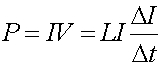This implies (by a little calculus) that the energy stored in an inductor isAs an example, we can derive the inductance of a solenoid. The magnetic field inside a solenoid of length l is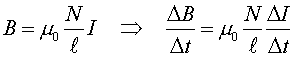as found previously. The flux through each turn is equal to B times the area A of the turn. The voltage across the solenoid is given by the rate of change of this flux times the number of loops: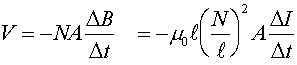Therefore, the inductance L can be given in terms of the geometry of the solenoid.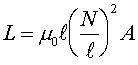Longer solenoids and solenoids with more cross-sectional area have more inductance. The strongest dependence is on the number of turns per unit length. One can also increase the inductance by using iron instead of a vacuum; in that case, the parameter m0 would be replaced by a larger value m .

Examples       Induction index        Lecture index